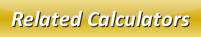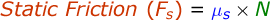# Static Friction Calculator

The static friction is a type of friction which occurs in-between two or numerous solid surfaces which doesn't move relative to each other.

Formula to calculate static friction is given by:where,
Fs = Static Friction [N]
μs = Coefficient of Static Friction
N = Normal Force [N]

In our below online static friction calculator, enter the coefficient of static friction and normal force in the input boxes and click calculate to static friction.

 Coefficient of Static Friction (μs): Normal Force (N): [N] Static Friction (Fs): [N]

Latest Calculator Release

Average Acceleration Calculator

Average acceleration is the object's change in speed for a specific given time period. ...

Free Fall Calculator

When an object falls into the ground due to planet's own gravitational force is known a...

Torque Calculator

Torque is nothing but a rotational force. In other words, the amount of force applied t...

Average Force Calculator

Average force can be explained as the amount of force exerted by the body moving at giv...

Angular Displacement Calculator

Angular displacement is the angle at which an object moves on a circular path. It is de...